# Excel If function examples, include if statement nest and combine multiple conditions with And(*)/Or

Lionsure 2019-10-26 Original by the website

The If function is one of the many functions used in Excel. It is used for logical judgment. The If function has three parameters, the first one is a condition, two or three are return values. If the condition is true, the second parameter is returned, otherwise return the third parameter.

The If function can be nested, and up to 64 If can be nested in an If function to achieve multiple conditions. In addition to combining multiple conditions with nesting, it is also possible to use the And function (or *) combination to represent multiple conditions of the AND relationship, and to combine OR conditions (or +) to represent multiple conditions of the OR relationship. You can also use array as a condition for the If function, which is often used in combination with other functions, such as the VLookUP function.

### I, Excel If function syntax

1. Expression: IF(Logical_Test, Value_If_True, [Value_If_False])

2. Description:

A. Logical_Test and Value_If_True are required, [Value_If_False] is optional. Optional options can be omitted, and must be enclosed in []. If the Value_If_True are omitted, a comma must be added after Logical_Test, otherwise the formula is wrong.

B. If function can nest up to 64 If, but as little as possible nesting, on the one hand is easy to read, on the other hand, the execution efficiency is also higher.

C. The If function can be used in combination with an array. If the array is used as an argument to the If function, when executed, each element of the array is taken out in order for calculation.

### II, The basic use of the If function in Excel

(I) Example 1: The if function has only one condition

1. Select cell E2, enter the formula =if(d2>500,), press Enter, return FALSE; double-click cell E2, change the greater than to less than, press Enter, return 0; the process steps, such as Figure 1 shows: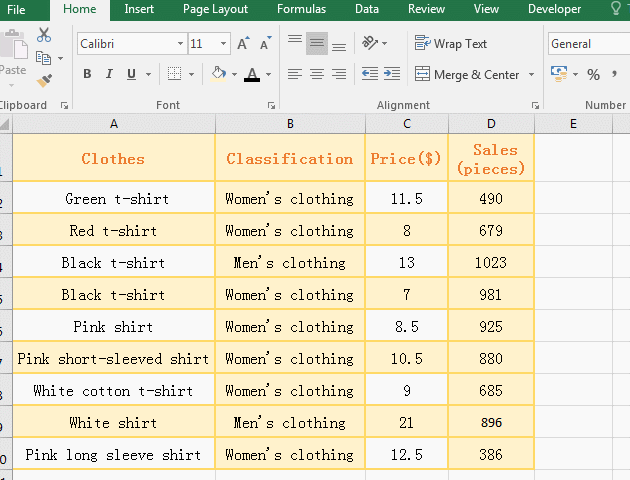Figure 1

2. When the If function has only one condition, the condition cannot omit the comma, such as the formula in the demo =if(D2>500,); when the condition D2>500 is false, it returns False; when the condition D2<500 is true , returns 0.

(II) Example 2: There are two and three parameters in the If statement

1. Select the E2 cell, copy the formula =IF(D2>=600,"Reach the standard"), and paste it in cell E2, press Enter, return FALSE; double-click E2, change the formula to =IF(D2>=600,"Reach the standard","Not up to standard"), press Enter, return to "Not up to standard"; select E2 again, move mouse to the cell fill handle that is in the lower right corner of E2, after the mouse becomes the plus sign, double-click the left button to return to the remaining clothing Sales evaluation results; operation process steps, as shown in Figure 2: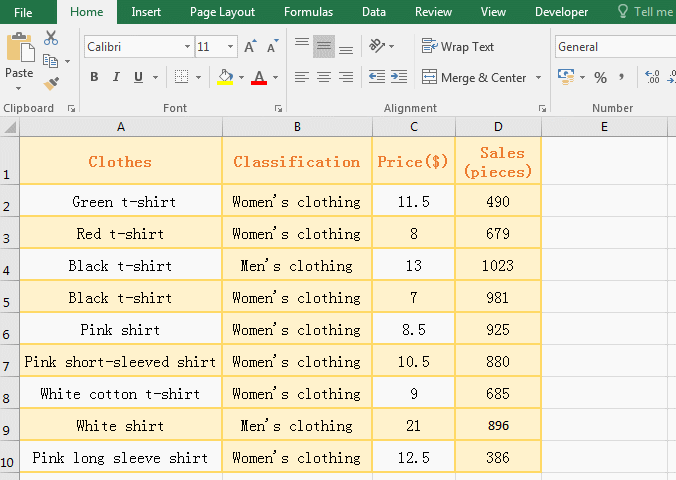Figure 2

2. The formula =IF(D2>=600, "Reach the standard") omits the last parameter, the second parameter does not need to add a comma, and the first parameter is added a comma, they are differet; when the condition D2>=600 is false, since the return parameter when the condition is false is omitted, FALSe is used instead. When the third parameter is added, and the condition D2>=600 is false, the third parameter is returned, that is, the "non-compliance" is returned.

### III, Excel If function multiple conditions nested use examples

(I) Nest a condition(Excel if statement)

1. If the sales of the clothing is greater than or equal to 900, it reach the standard, otherwise it don't reach the standard. Now it is required to find out the women's clothing that reach the standard.

Select cell E2 and copy the formula =IF(B2="Women's clothing",IF(D2>=900,"Reach the standard","Not up to standard"),"Not up to standard"), and paste it in cell E2, press Enter, return to "Not up to standard"; select cell E2 again, move the mouse to the cell fill handle in the lower right corner of E2, after mouse becomes the black plus sign, double-click the left button to return the evaluation of the remaining clothing; the operation steps are as shown in Figure 3: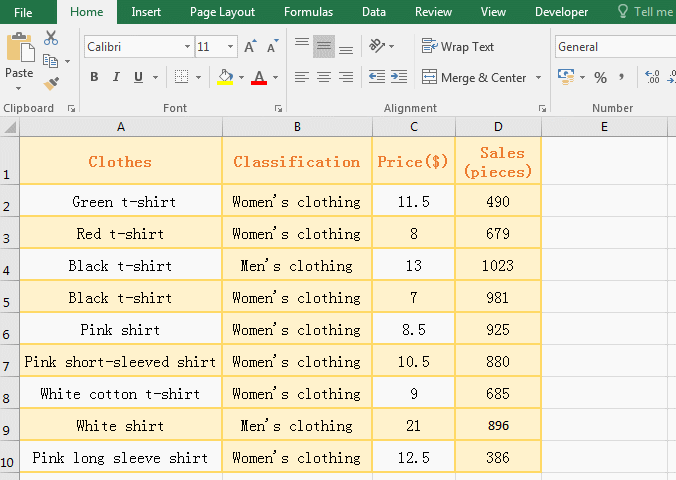Figure 3

2. Formula description:

A. Formula =IF(B2="Women's clothing",IF(D2>=900,"Reach the standard","Not up to standard"),"Not up to standard") Nested an IF statement, the implementation process is: first take condition of the outer IF B2="women's clothing" to judge, if it is true, execute nested IF statement IF(D2>=900,"Reach the standard","Not up to standard"); otherwise return "Not up to standard" of outer IF, ie return "Not to standard" that is at the end of the formula.

B. When executing a nested IF statement IF(D2>=900,"Reach the standard","Not up to standard"), first take the condition D2>=600, if it is true, return "Reach the standard", otherwise return "Not up to standard".

(II) Nest two conditions(Excel if statement)

1. If you want to find the women's clothing with the sales greater than or equal to 900 and the price less than \$10, return to "Reach the standard" if qualified, otherwise return "Not up to standard".

Select cell E2 and put the formula =IF(B2="Women's clothing",IF(D2>=900,IF(C2<10, "Reach the standard","Not up to standard"),"Not up to standard"), "Not up to standard") copy to E2, press Enter, return to "Not up to standard"; also use the method that is the cell fill handle by double-clicking to return whether the other clothing meet the standard; the operation steps are as shown in Figure 4: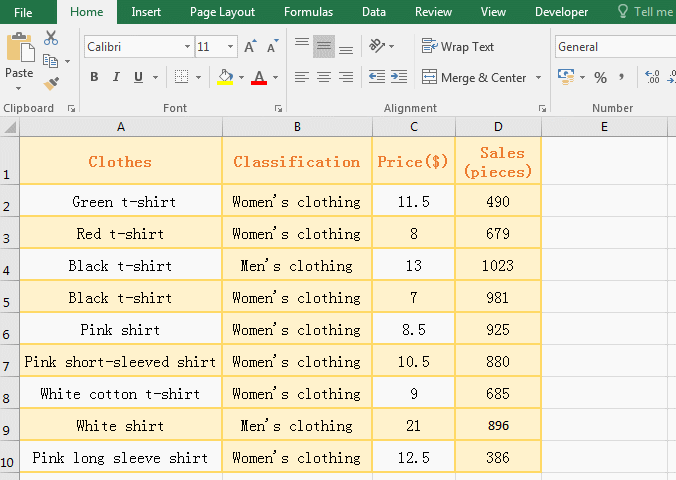Figure 4

2. Formula description

A. Formula =IF(B2="Women's clothing",IF(D2>=900,IF(C2<10, "Reach the standard","Not up to standard"),"Not up to standard"), "Not up to standard") nested two IF statements; when the formula is executed, the condition B2="Women's clothing" of the outermost IF is first taken out, if it is established, the first nested IF statement is executed, that is, IF(D2>=900,IF(C2<10, "Reach the standard","Not up to standard"),"Not up to standard"); otherwise return to "Not up to standard" of the outermost IF.

B. When executing the first nested IF statement, the condition D2>=900 is also taken out first; if it is established, the second nested IF statement is executed, that is, IF(C2<10, "Reach the standard","Not up to standard"); otherwise returns "Not up to standard" of the first nested IF statement.

C. When executing the second nested IF statement, the condition C2<10 is also taken out first; if it is established, it returns "Reach the standard", otherwise it returns "Not up to standard". if there are more Ifs in the nest, the same is also execute in this order.

### IV, If formula in excel uses And(*) or OR(+) to combine multiple conditions.

(I) Combining multiple conditions with And(*)

1. Similarly, to find the women's clothing with the sales greater than or equal to 900 and a price less than \$10, return to "Reach the standard" if qualified, otherwise return "Not up to standard" as an example.

Select cell E2 and copy the formula =IF(AND(B2="Women's clothing", D2>=900, C2<10), "Reach the standard", "Not up to standard") to E2, press Enter, return to "Not up to standard"; also use the same method that is the cell fill handle by double-clicking to returns the evaluation of the remaining clothing. Delete the contents of E2:E10, double-click E2, and copy the formula =IF((B2="Women's clothing")*(D2>=900)*(C2<10),"Reach the standard","Not up to standard") to E2; press Enter, return to "Not up to standard", and return the evaluation of the remaining clothing by double-clicking the cell fill handle. The results returned by the two formulas are the same. the operation steps are as shown in Figure 5: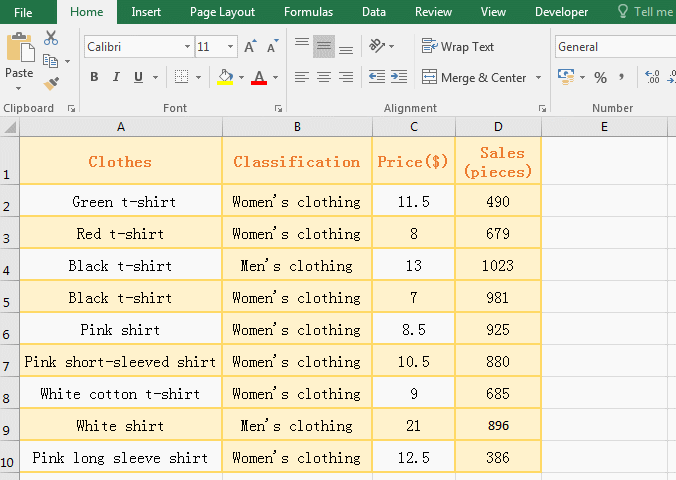Figure 5

2. Formula description

1. The conditions of formula =IF(AND(B2="Women's clothing", D2>=900, C2<10), "Reach the standard", "Not up to standard") are AND(B2="Women's clothing", D2>=900, C2<10), that is, use the And function to combine three conditions, each condition is separated by a comma. When only three conditions are true, And funtion returns true, otherwise it returns false; when And function returns true, the IF statement returns "Reach the standard, otherwise IF statement returns "Not up to standard".

2. In addition to the And function can be used to combine condition that indicates the "AND", you can also use the asterisk * combination, each condition should be enclosed in parentheses, and the condition and condition are connected with *, such as the conditions in the demonstration (B2="Women's clothing")*(D2>=900)*(C2<10), which is equivalent to AND(B2="Women's clothing", D2>=900, C2<10).

(II) Combine multiple conditions with OR(+)

1. If you want to mark clothing with sales less than 400 or greater than or equal to 800 or a price greater than \$10. Select cell E2 and copy the formula =IF(OR(D2<400,D2>=800, C2>10), "Satsfied","Not satisfied") to E2, press Enter, return "satisfied"; returns whether the remaining clothing satisfy the condition by double-clicking the filling handle of the cell; the operation process steps are as shown in Figure 6: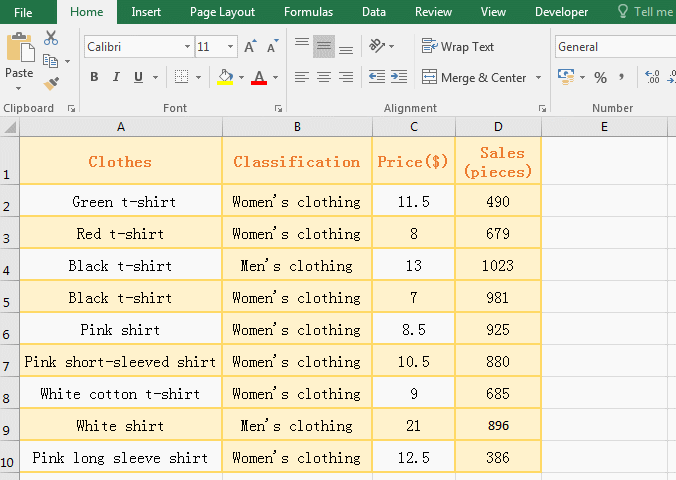Figure 6

2. Formula description

The conditions of the formula =IF(OR(D2<400,D2>=800, C2>10), "Satsfied","Not satisfied") is OR(D2<400,D2>=800, C2>10), which is the relationship of "or" means that true is returned as long as one condition is met; if OR(D2<400,D2>=800, C2>10) returns true, IF statement returns "Satisfied", otherwise IF statement returns "Not satisfied".

3. Use plus sign + instead of OR to combine the conditions of the "OR" relationship

Put the formula =IF(OR(D2<400,D2>=800, C2>10), "Satsfied","Not satisfied")

Use the plus sign + instead of OR to become:

=IF((D2<400)+(D2>=800)+(C2>10), "Satisfied", "Not satisfied")

The results returned by these two formulas are the same.